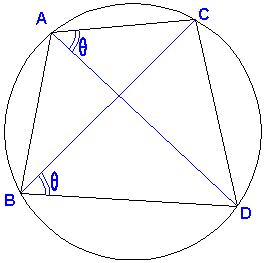# Ptolemy via Cross-Ratio

### Mariano Perez de la Cruz September 9, 2011

Recollect two well known properties of the cross-ratio.

1. Given four points on a plane if cross-ratio value (ABCD) = m, them the cross-ratio value, obtained by permutation the two interior points B and C, (ACBD) = 1 - m.
2. If complex numbers a, b, c, d represent points A, B, C and D, then the four points A, B, C, and D all belong to a same circle (or a straight line) iff the cross-ratio (abcd) is a real number.

For example, the cross-ratio (abcd) is the quotient of two single ratios (acd) = (a - c)/(b - c) and (bcd) = (a - d)/b - d). These single ratios are complex numbers La and Lb which may have different moduli, but always have same argument, provided the pairs of points {A, B} and {C, D} do not separate each other. Thus we see that

(acd) = (a - c)/(a - d) = (|a - c|/|a - d|) eθ = La,
(bcd) = (b - c)/(b - d) = (|b - c|/|b - d|) eθ = Lb.Indeed both arguments have the same value; we see the segment DC under the same angle θ either from A or B, as long as A, B, C and D are concyclic and A and B lay on the same side of segment CD. Therefore the quotient La/Lb of the two numbers La and Lb with the same arguments is real and equal to the ratio of their moduli, |La|/|Lb|. As a consequence, (ABCD) = (abcd), whenever points A, B, C, D are concyclic (or collinear) and the pairs {A, B} and {C, D} do not separate each other. In addition, in all eligible cases, (ABCD) = m > 0. It is convenient to order the points as in the above diagram.

The same procedure applies to the other cross-ratio (ACBD), and we see that also (ACBD) = (acbd). (So, too, (ACBD) = 1 - m > 0, implying in passing that 0 < m < 1.)

Ptolemy's theorem is just a direct consequence of the above and is equivalent to

(ABCD) + (ACBD) = 1.

Indeed, if we express the sides and the diagonals of the quadrilateral ABCD as the moduli of the related complex numbers: |a - c| = AC, |a - d| = AD, and so on, then (ABCD) + (ACBD) = 1 expands to